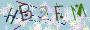## 一个PHP在线编辑服务端指定TXT文本的实例

2015-04-27 浏览:2702```<?php
function get_txt(\$robots_file)
//定义函数，内容用{}括起来
{
if(file_exists(\$robots_file))
//如果文件存在，读取其中的内容
{
\$fp=@fopen(\$robots_file,"r");
if (\$fp) {
while (!feof(\$fp)) { //如果没有到文件尾部
\$robots_txt = fgets(\$fp, 4096); //逐行读取
\$robots_all = \$robots_all.\$robots_txt; //将数据保存到\$robots_all里面
}
return(\$robots_all); //返回所有内容
fclose(\$fp); //关闭文件
}
}
}

function put_txt(\$robots_txt)
{
\$fp=fopen("robots.txt","w");
//w是write的缩写，代表写入的意思，以写入的方式打开文件
fputs(\$fp,\$robots_txt);
//输出文本到文件
fclose(\$fp);
}
?>

<?php
\$edit=\$_GET["edit"];
\$txt=\$_POST["txt"];
\$get_txt=get_txt("robots.txt");
//调用刚才定义的函数打开robots文件。

if(\$edit=="write")
{
put_txt(\$txt);
echo "成功保存 <a href=robots-editer.php>返回</a>";
}
else
{
echo "成功读取<a href=robots.txt target=_blank>robots.txt</a> <a href=writer.php>返回</a>";
}
?>

<?php
if(\$edit=="")
{
?>
<form name="form1" action="?edit=write" method="post">
<textarea name="txt" cols="160" rows="30"><?php echo \$get_txt; ?></textarea>
<br />
<input name="submit" value="保存" type="submit" />
</form>
<?php
}
?>```

#### 发表评论:◎欢迎参与讨论，请在这里发表您的看法、交流您的观点。